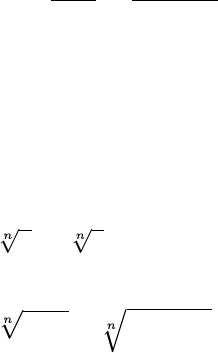Class Notes (1,100,000)
CA (620,000)
U Windsor (2,000)
55 (300)
Lecture 1

# 55-140 Lecture Notes - Lecture 1: Rational NumberExam

Department
Biological Sciences
Course Code
BIOL 1111
Professor
Animesh Sarker
Lecture
1

This preview shows half of the first page. to view the full 3 pages of the document.Diﬀerential Calculus
MTH 62-140
1. Suppose cis a constant and the limits lim
xa
f(x) and lim
xa
g(x) exist.
Then
(a) lim
xa
[f(x) + g(x)] = lim
xa
f(x) + lim
xa
g(x)
(b) lim
xa
[f(x)g(x)] = lim
xa
f(x)lim
xa
g(x)
(c) lim
xa
cf(x) = clim
xa
f(x)
(d) lim
xa
[f(x)·g(x)] = lim
xa
f(x)·lim
xa
g(x)
(e) lim
xa
f(x)
g(x)=
lim
xa
f(x)
lim
xa
g(x)if lim
xa
g(x)6= 0
2. lim
xa
[f(x)]n= [lim
xa
f(x)]nwhere nis a positive integer.
3. lim
xa
c=cand lim
xa
x=a
4. lim
xa
xn=anwhere nis a positive integer
5. lim
xa
n
x=n
awhere nis a positive integer. If nis even, we assume
that a > 0.
6. lim
xa
n
pf(x) = n
qlim
xa
f(x) where nis a positive integer. If nis even, we
assume that lim
xa
f(x)>0.
7. Direct Sustitution Property: If fis a polynomial or a rational function
and ais in the domain of f, then
lim
xa
f(x) = f(a)
8. If f(x) = g(x) when x6=a, then lim
xa
f(x) = lim
xa
g(x), provided the
limits exist.